# M8-S9: Strong Nuclear Force and Radioactive Decay (Theory)

﻿

### What keeps the nucleus together?

• Electrostatic repulsion and gravitational attraction between masses in the nucleus are two forces that act between nucleons.
• When quantitatively comparing the forces, it is seen that the electrostatic forces which push the nucleons apart is far greater than the gravitational attraction between the nucleons.
• In fact, the gravitational attraction between them is so small it can be disregarded in most calculations involving the forces between nucleons.
• From the calculations below, it can be seen that electrostatic repulsion vastly overcomes gravitational attraction between nucleons.Strong Nuclear Force

• The electrostatic repulsion between protons is significantly overcome by strong nuclear force between nucleons (protons and neutrons)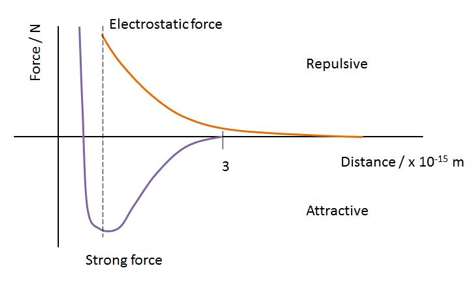• Strong nuclear force is flexible in nature:
• It is attractive when nucleons are in normal distance from each other. This attractive force decreases with distance.
• It is repulsive when nucleons are close together.
• It is stronger in magnitude and opposite in direction to electrostatic force at typical nucleon separation.• Strong force acts between nucleons separated by small distances while electrostatic force affects them over a wide range of distances.
• The nature of forces present in the nucleus determines nuclear stability and is the main cause of radioactive decay.

Nuclei are naturally unstable because:

1. Large nuclei - As the number of nucleons in a nucleus increases, the distance between them inevitably becomes smaller. This causes the strong nuclear force to become more frequently repulsive. When the atomic number (Z) is greater than 83, the electrostatic repulsion between protons becomes so immense that almost all isotopes are unstable.
1. Too many protons – Protons contribute to both strong nuclear and electrostatic force. However, as the number of protons increases, the distance among them inevitably also increases. Since electrostatic repulsion dominates over strong force’s attraction over long distances, the net force between protons is repulsive for large nuclei (too many protons).
1. The neutron to proton ratio is unstable
• For nuclei with an atomic number less than or equal to 20, stable isotopes have a n/p ratio of 1.
• As a nucleus becomes larger, the stable n/p ratio becomes greater than 1. This is because more neutrons are required to overcome the stronger electrostatic repulsion between greater number of protons.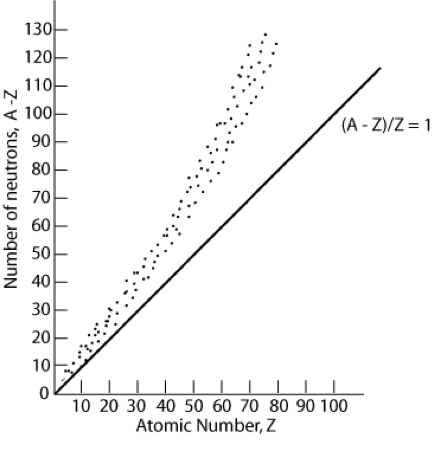A is the atomic mass and Z is the atomic number (number of protons). Linear line shows a one-to-one ratio between neutrons and protons

• This instability results in radioactive decay in the atom’s attempt to reach a more stable state.
• In nuclear physics or chemistry, 'atom' is often replaced by the more precise term 'nuclide'. Nuclides are atomic species with specific number of neutrons and protons.
• An isotope is a species with different number of neutrons but same number of protons. It is usually used to compare nuclides of the same element.
• A radioactive isotope is an isotope with an unstable nucleus.
Radioactive decay can happen in four ways:
• Alpha (α) decay – The nucleus emits an alpha particle (helium nucleus). It consists of two protons and two neutrons. This will move the atom two elements up in the periodic table as it has lost two protons, for example: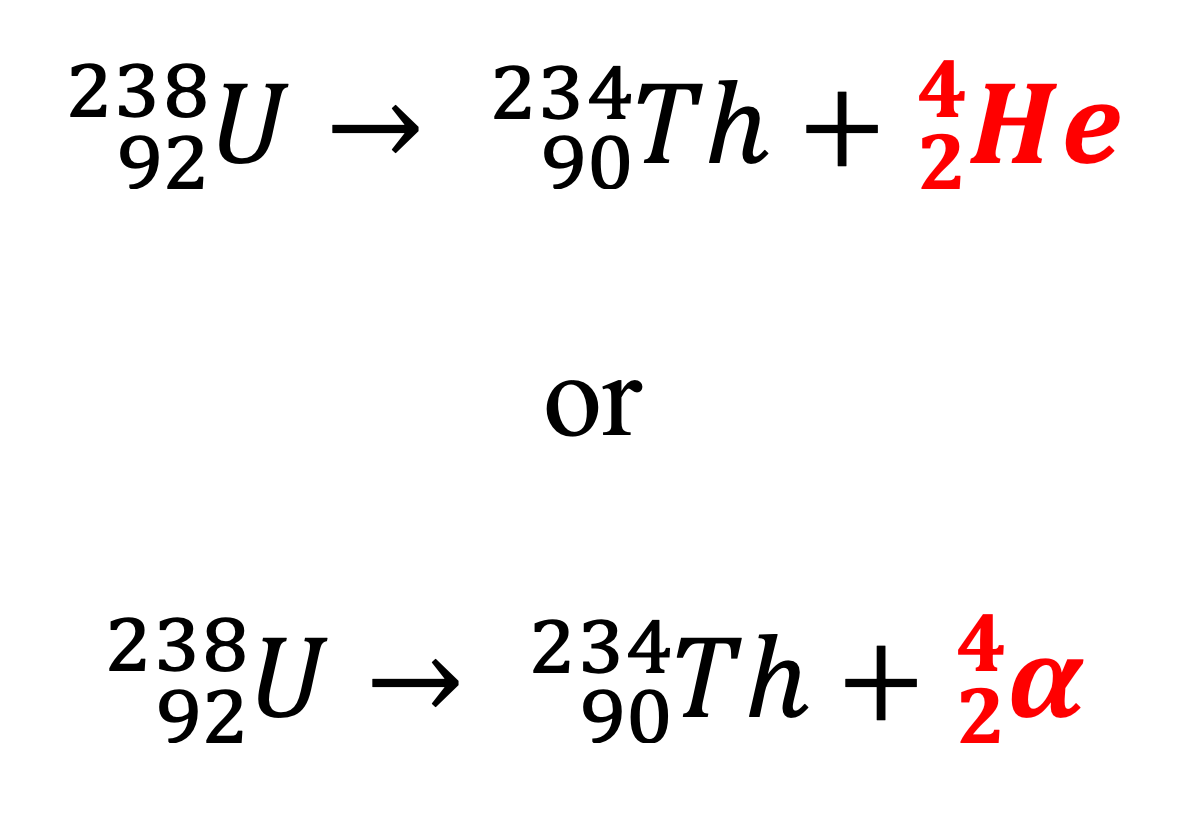• Beta-minus (β-) decay – This involves the decay of a neutron into a proton which stays in the nucleus, raising the atomic number by 1 while keeping the atomic mass number constant. An electron and an antineutrino are emitted: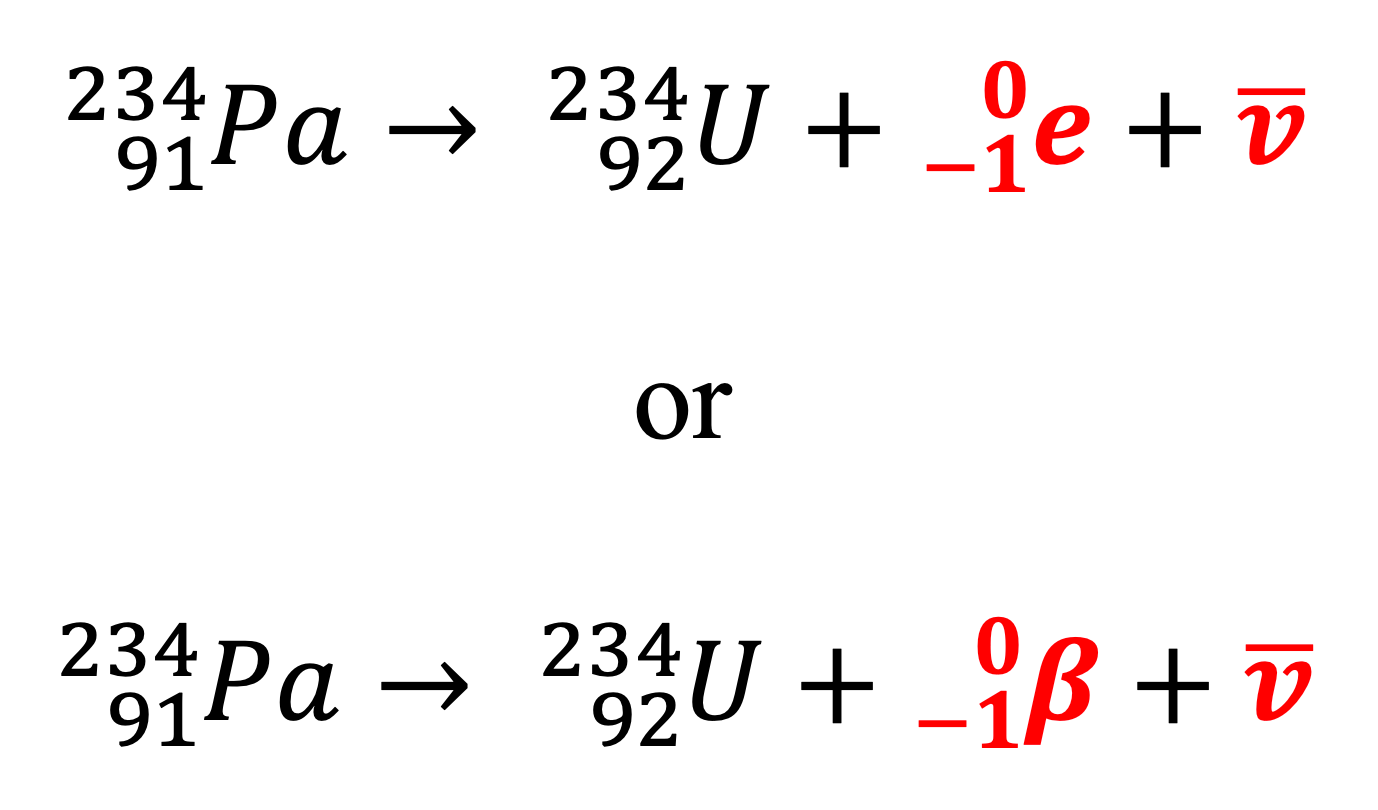• Beta-plus (β+) decay – This involves the decay of a proton into a neutron which stays in the nucleus, reducing the atomic number by 1 while keeping the atomic mass number constant. A positron (antiparticle of electron) and a neutrino are emitted:• Gamma (γ) decay – This involves the release of gamma photons to allow nuclides to reach a lower energy state. Gamma decay usually occurs after alpha and beta decays as they produce nuclides in excited states. Nuclides in excited states return to their ground state through emission of gamma photons.For example, cobalt-60 undergoes beta-minus decay to form nickel-60 while emitting an electron and an antineutrino in the process.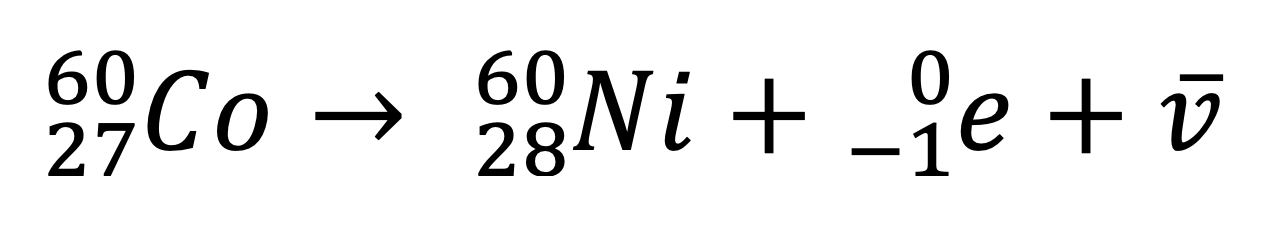The nickel-60 nuclide is currently in an excited state and will emit a gamma photon of 1.17 MeV of energy to reach a lower energy state.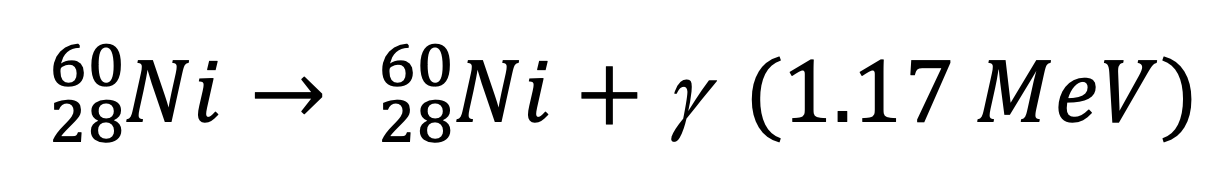This is followed by another gamma decay (emission of 1.33 MeV photon) to reach the ground state.Sometimes, the first stage of gamma decay is absent as cobalt-60 can emit a beta particle of higher energy (happens 0.12% of the time).

### Wilson’s Cloud Chamber

• The Cloud chamber uses the ionising properties of radiation in order to detect it within the apparatus. Inside the chamber is alcohol vapour which is colder towards the bottom.• This vapour is supersaturated at the bottom, so it is more likely to condense.
• An electric field is applied to clear the cloud chamber of any stray ions which could cause vapour to condense around them.
• When alpha and beta radiation pass through the chamber, they ionise air particles, and alcohol condenses around them forming a trail.
• The type of radiation can be determined by observing the vapour trails formed.
• Alpha radiation - A short, thick vapour trail is formed from alpha radiation. This is because alpha particles have high mass and a larger positive charge letting it ionise the particles easier.
• Beta radiation - Beta particles on the other hand, move faster and have less mass – thus they are not as ionising as alpha particles. This means that they vapour trails indicative of beta emission will be thinner and more intermittently formed.
• Gamma radiation - While gamma radiation (photons) are neutral in charge, they are indirectly ionising. They can remove electrons from atoms through the photoelectric and Compton effect. However, it is difficult for gamma photons to ionise air particles than metals as electrons are delocalised in metals. As a result, gamma radiation is usually not observed in the Cloud Chamber.

 Properties Alpha Decay/Radiation Beta-minus Decay Beta-plus Decay Gamma Decay/radiation Cause Radioisotope is too large, contains too many nucleons n/p ratio is too high n/p ratio is too low Relaxation of excited nuclides Emission Helium nucleus Electron and antineutrino Positron (and neutrino Gamma photon Charge Positive Negative Negative Neutral Electric & Magnetic Fields Small deflection Large deflection opposite to alpha particle due to large q/m ratio Large deflection due to large q/m ratio. Same direction as alpha particle No Interaction Ionising Ability Strong Moderate Moderate Very weak and indirect (photoelectric and Compton’s effect) Penetrating Power Very weak, can be stopped by a sheet of paper Moderate, stopped by a sheet of thin aluminium foil Moderate, stopped by a sheet of thin aluminium foil Very strong, stopped by at least 10 cm of lead (dense material) Observation in Wilson’s Cloud Chamber Clear and defining streaks Thinner and less visible streaks compared to alpha radiation Thinner and less visible streaks compared to alpha radiation Usually difficult to see or absent

Practice Question 1
(a) Explain how the strong nuclear force changes with distance between nucleons. (2 marks)
(b) Compare the strong nuclear force and electrostatic force that exist in the nucleus of an atom. (3 marks)

Practice Question 2
Aluminium-27 nucleus is an unstable nucleus that commonly undergoes alpha decay.
(a) Explain why aluminium-27 nucleus is unstable. (1 mark)
(b) What products are formed as a result of this alpha decay? (1 mark)

Previous section: de Broglie and Schrodinger's Contribution to Modern Physics

Next section: Modelling Radioactive Decay (Calculation)